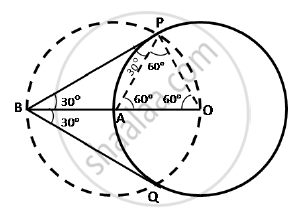# Draw a circle of radius 4 cm. Draw two tangents to the circle inclined at an angle of 60° to each other. - Mathematics

Draw a circle of radius 4 cm. Draw two tangents to the circle inclined at an angle of 60° to each other.

#### Solution

Steps of construction:

(i) Take a point O on the plane of the paper and draw a circle of radius OA = 4 cm.

(ii) Produce OA to B such that OA = AB = 4 cm.

(iii) Draw a circle with centre at A and radius AB.

(iv) Suppose it cuts the circle drawn in step (i) at P and Q.

(v) Join BP and BQ to get the desired tangents.Justification:

In OAP, OA = OP = 4 cm ...(radii of the same circle)

Also, AP = 4 cm ….(Radius of the circle with centre A)

∴ ΔOAP is equilateral.

∴ ∠PAO = 60°

∴ ∠BAP = 120°

In BAP, we have BA = AP and ∠ BAP = 120°

∴ ∠ABP = ∠APB = 30°

Similarly we can get ∠ABQ = 30°

∴ ∠PBQ = 60°

Concept: Construction of Tangents to a Circle
Is there an error in this question or solution?
2015-2016 (March) All India Set 1

Share